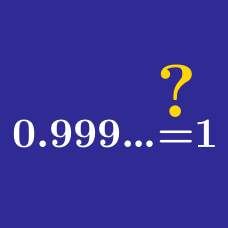Algebra

# Algebra Common Misconceptions: Level 1 Challenges

$\large \sqrt{4} = \, \color{#D61F06}{?}$

$\LARGE 2^{2^{2^{2^0}}} = \ ?$

$\large A = \frac{1}{\left(\frac{0.5}{0.25}\right)} \hspace{5mm} \text{or} \hspace{5mm} B = \frac{\left(\frac{1}{0.5}\right)}{0.25}$

Which of these numbers above is larger?

True or False?

$0.9999 \ldots = 1$


Note: The "$\ldots$" indicates that there are infinitely many 9's.

$\large (a-b) - c = a - (b - c)$

Suppose $a, b$ and $c$ are numeric variables. Under what conditions is the equation above true?

×